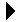Rational Number Example Client# Rational Number Example ClientRational Number Package Body Download Linked Stack Package Specification```--
-- Simple test driver for the Rational_Number package.
--
with Gnat.Io; use Gnat.Io;
with Rational_Number; use Rational_Number;
procedure RatTest is
R: Rational := To_Rational(6, 15);
R2: Rational := To_Rational(2);
R3: Rational;
begin
R3 := To_Rational(5,7) * (To_Rational(4,9) + R) / R2;
Put("The answer is: ");
Put(R3);
New_Line;

if R3 = To_Rational(65, 112) then
Put_Line("Yes");
else
Put_Line("No");
end if;

if R3 = To_Rational(114,378) then
Put_Line("Yes");
else
Put_Line("No");
end if;
end RatTest;
```Rational Number Package Body Linked Stack Package Specification